• supervised learning(监督学习): 使用已经标注的数据计算出模型，regression/classification
• unsupervised learning(无监督学习): 使用没有具体标记信息的数据，计算机分类得到模型，cluster(聚类)

# Regression

## Linear Regression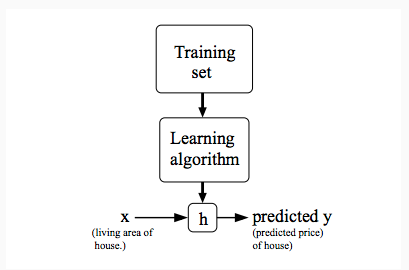cost function, squared error function, 其中1/2是因而计算方便而引入（求导的时候会乘以2）: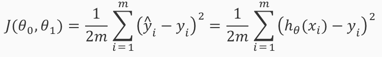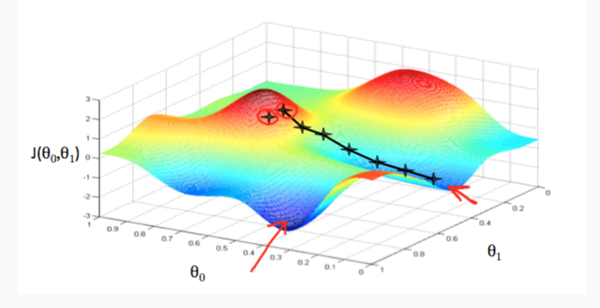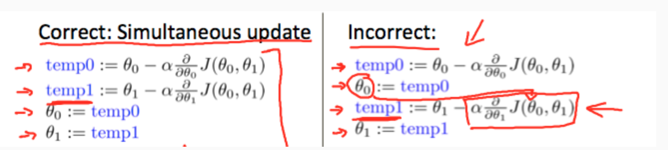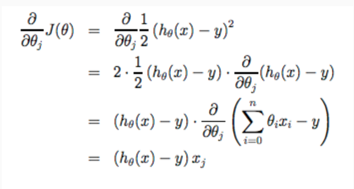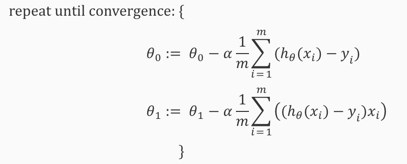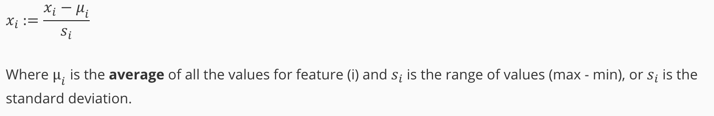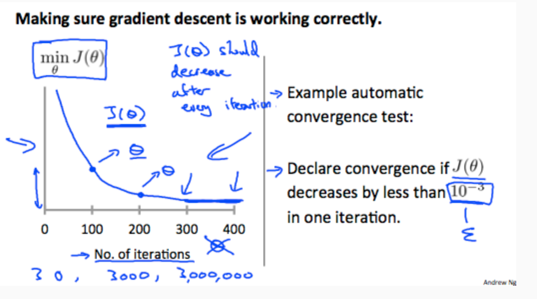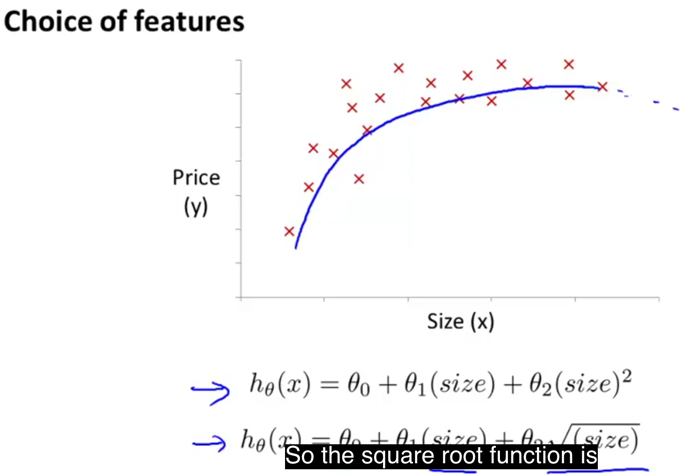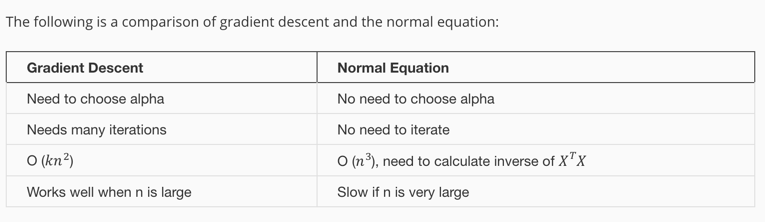$\theta = \theta - \alpha {1 \over m} X^T(X \theta - y)$

1. 将求和看做矩阵相乘。
2. 求和坐标就是矩阵相乘中间的维度（规约）。
3. 根据矩阵维度调整顺序，比如公式中$X$放在右边，计算矩阵时调到左边，且调为$X^T$

## Logistic Regression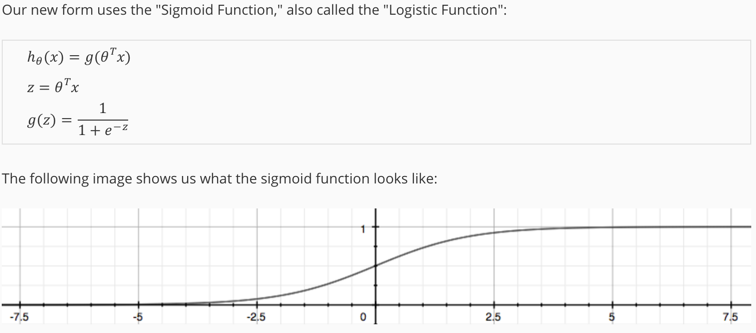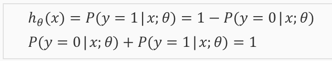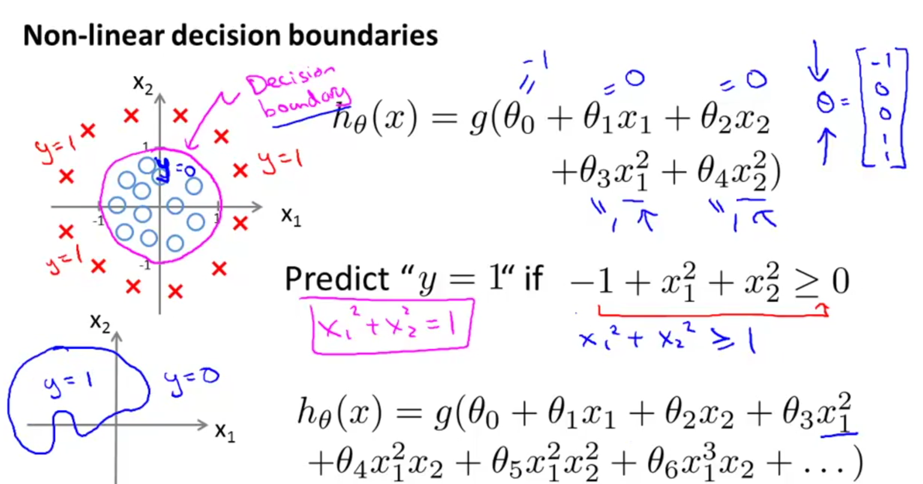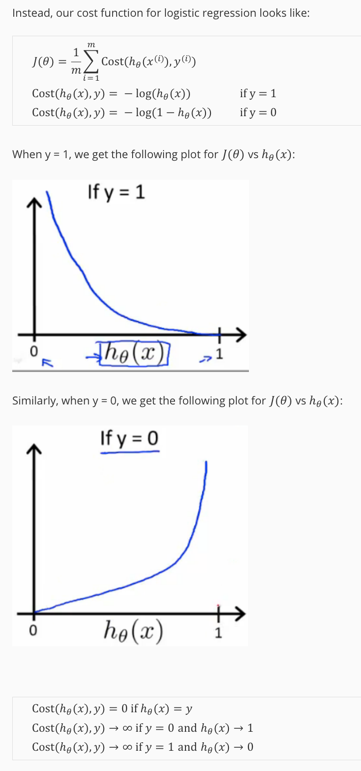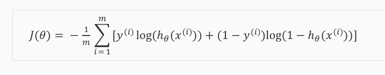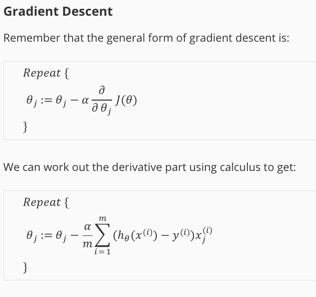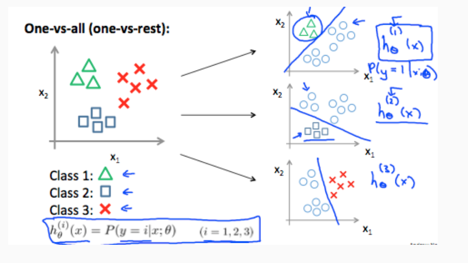1. 本质上都是优化问题，只是代价函数不同。
2. 通过数学的方式，将分类转换为概率，然后转换为代价，进而转为优化问题。

## Overfitting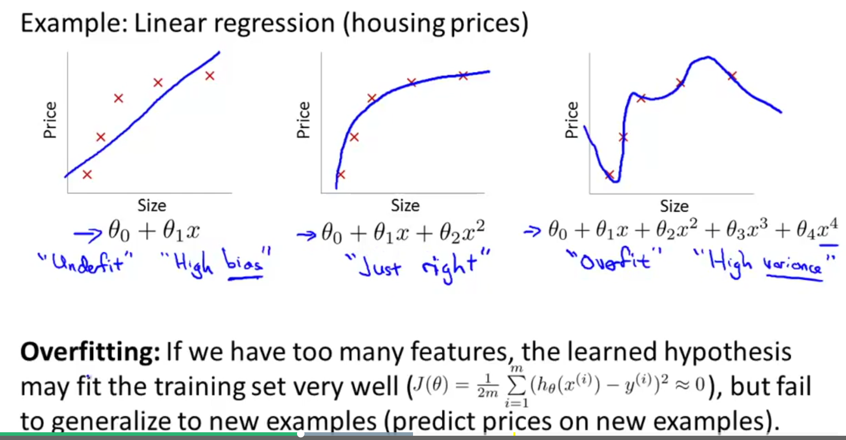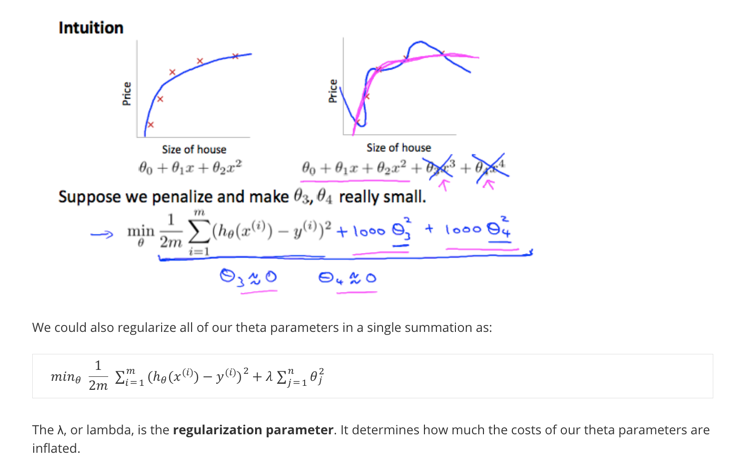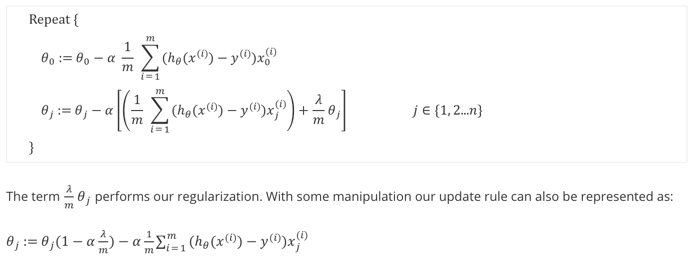# Neural Networks

1. 特征太多，无法进行计算。线性模型如果考虑多阶数据，计算量成次方级数增长。
2. 现实世界大多数问题不是线性。

## Concept

1. 引入层的概念。
2. 每一层的数据是上一层数据传导之后的激励(activation)
3. 最后一层输出结果。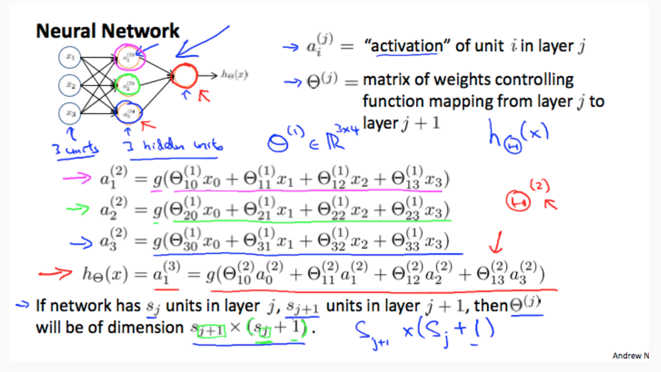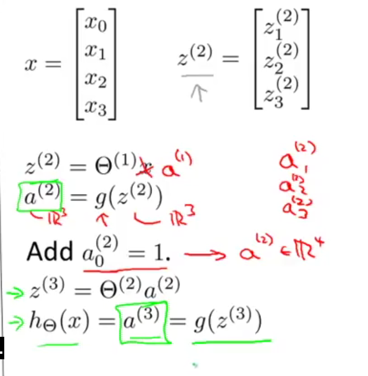1. 中间叫做隐藏层(hidden layer), 两边叫做输入层和输出层。
2. 每一层的第一个数据$a_0^j = 1$，叫做bias nodes
3. $z^j = \Theta^{j-1} a^{j-1}$ 表示每一层的$z$数值是上一层数据传导到新一层的结果。实际结果还需要logestic，就是$a^{(i)} = g(z^i)$
4. $\Theta^j$表示权重矩阵(weights matrix)，第$j$层的维度是$s_{j+1} \times (s_j + 1)$，计算方式是右乘数据，且要注意0号数据的存在。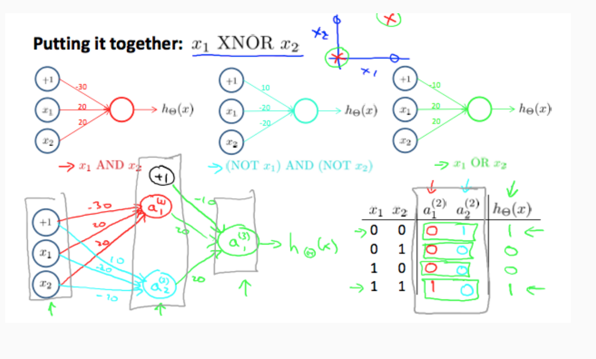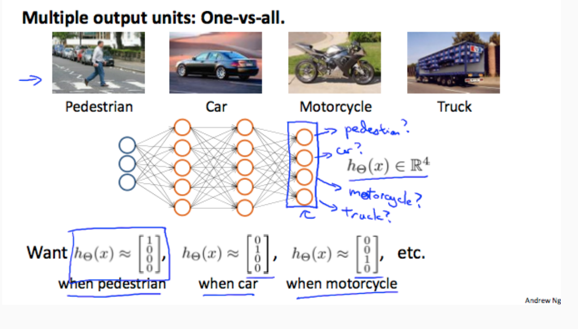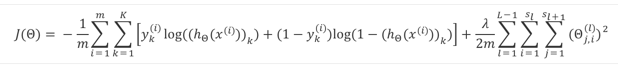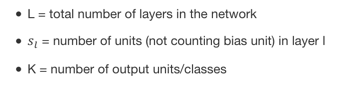## BP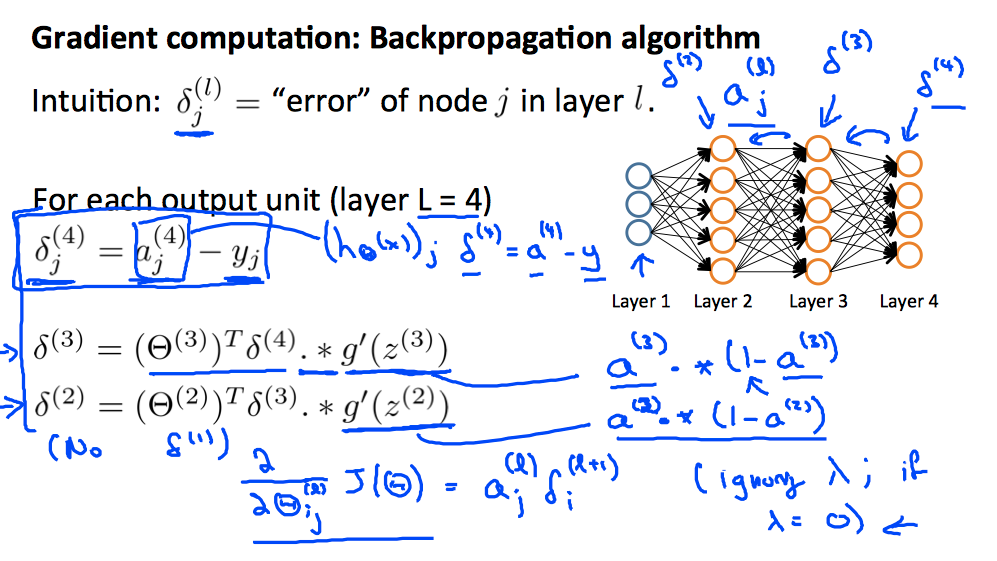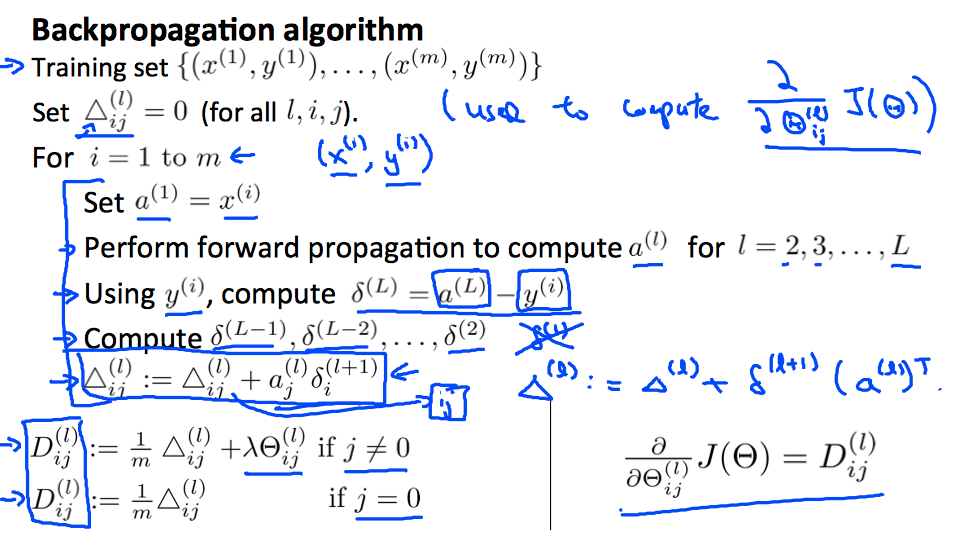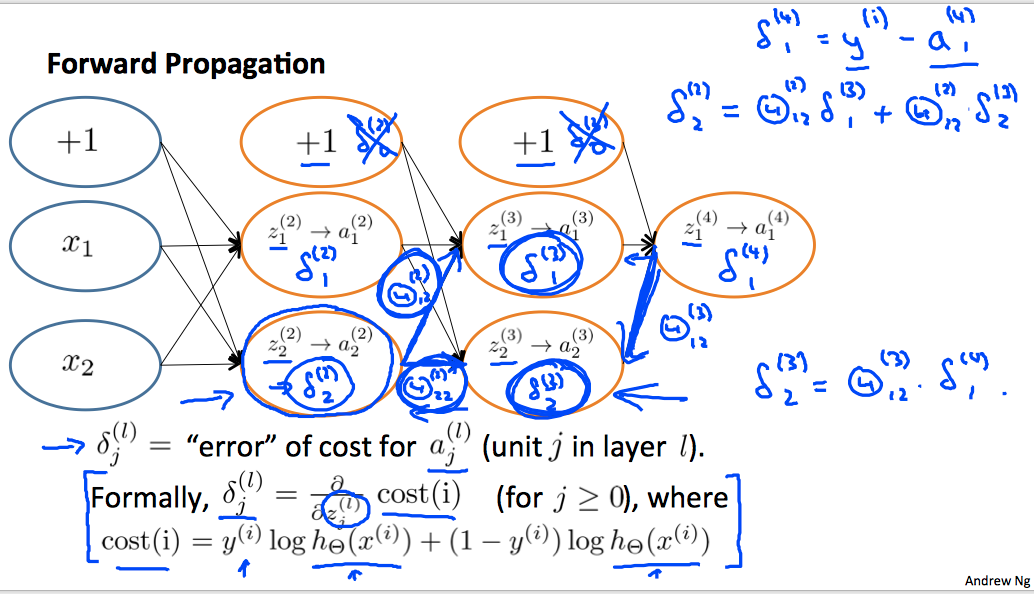1. 表示第$l$层第$j$元素的「误差」，这个误差实际含义是第$l$层的$cost(l)$对第$l-1$层没有logestic的$z^{(j-1)}$求导。
2. 或者换个说法，表示前一层的$z^{(l-1)}$如何变化，第$l$层的偏差越小。

## Implementation

• rolling/unrolling parameters

优化函数计算时，传入的$\theta$参数都是列数据，而我们这里的$\Theta$是多个矩阵构成的大数据，所以计算的时候，需要将矩阵压缩成向量。但是计算cost的时，再将矩阵还原回来，计算代价。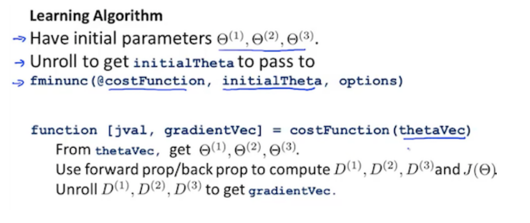• grandient checking。

为了验证BP算法实现正确，重点是求导函数计算正确，可以用数值计算方式求导，然后验证是否计算正确。注意，实际计算BP时需要去掉验证，因为性能代价大。之所以用解析方式求导就是因为数值计算方式太耗性能。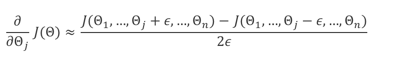• random initialization

初始参数不可以对称，如果对称，计算的中间结果都相同，得不到好效果。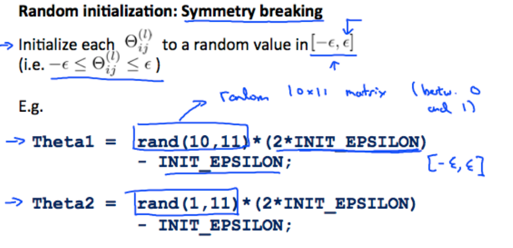课后作业中提到的一种比较好计算随机数范围的经验公式：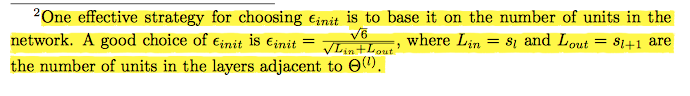• network architecture

隐藏层的节点个数要都一样，理论上隐藏层越多，效果越好，计算越多。

• wrap up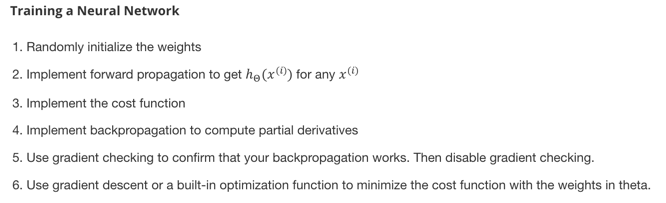1. 要经常想着维度的概念，脑子里面要知道维度是多少，然后才可以有效地计算矩阵相乘。

2. 和logestic regression计算形式不一样，logestic的多元判定是计算K次结果，每一次判定的目标向量y是一个m元的向量y == target_type。但神经网络计算时候没有多次计算，这要求每一行数据计算得到的y就是一个向量，且其中只有一个数据是1，逻辑是y = zeros(m, 1); y(target_type) = 0;

3. 一定要小心bias node。

计算反向误差$\delta$时，反向的结果考虑了bias node（正向计算输入层多一个节点，所以反向计算回来时也多一个），但这个数据并不用到，要小心。

还有就是计算regularization时不考虑bias node，也需要去掉：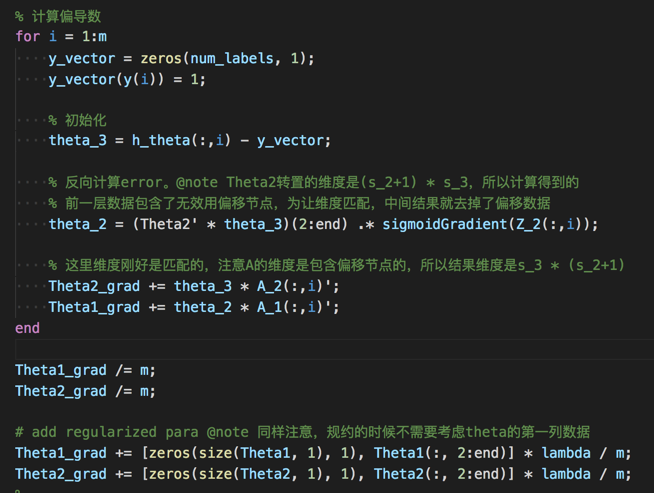# Evaluating & Improve

## Evaluating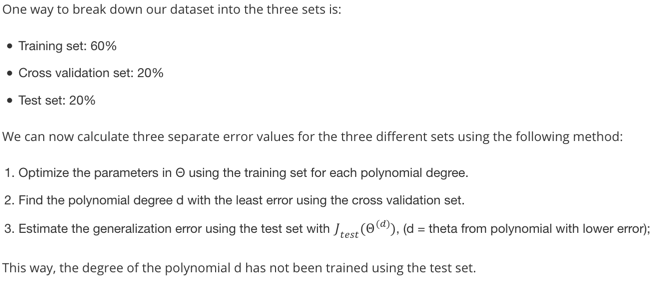## Bias vs Variance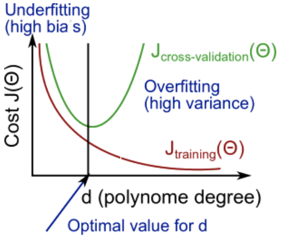• $J_{train}^{(\Theta)}$$J_{CV}^{(\Theta)}$都很高，且数值接近时，underfit。这时候你训练的结果既不能拟合数据，也不能预测未知数据。
• $J_{train}^{(\Theta)}$很小，但 $J_{CV}^{(\Theta)}$很大，且远大于$J_{train}^{(\Theta)}$的时候，overfit。这时候你训练的结果可以拟合数据，但不能预测未知数据。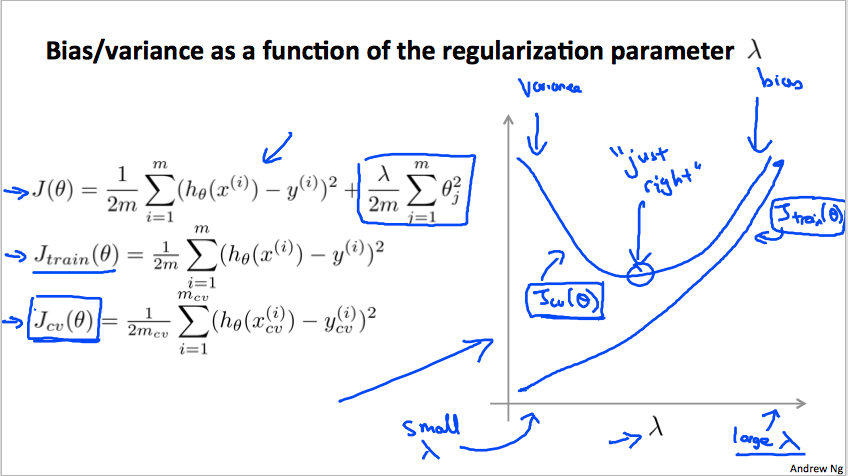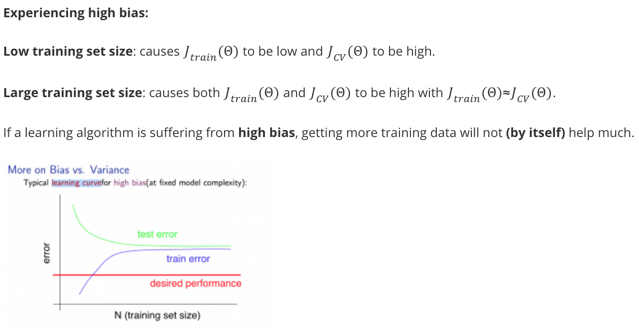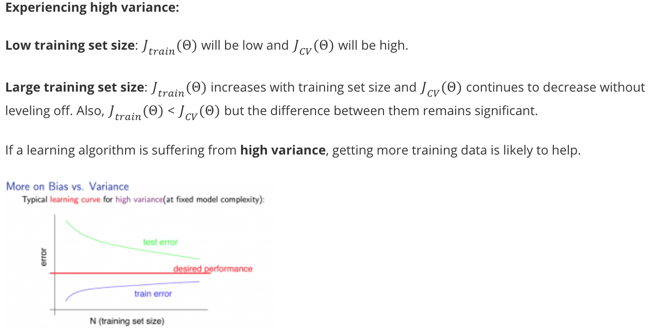1. 如果underfit，两个集合的误差都很大，数值也接近，数据越多并不能得到更好效果（因为压根没有办法拟合）
2. 如果overfit，两个集合的误差间隔较大，数据越多，效果会越好（因为高阶特性能更好的得到整体考虑，进而拟合出更好的模型。）

method fix
Get more training examples fix overfit
Try smaller sets of features fix overfit
Try get addional features fix underfit
Try adding polynomial features fix underfit
Try increase $\lambda$ fix overfit
Try decrease $\lambda$ fix underfit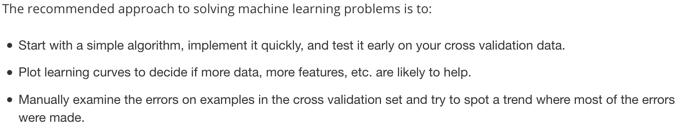• 选择足够多的特征（logestic/linear regression），或者神经网络使用足够多的hidden units（神经网络的隐藏层就是在扩充特征的数目，而且是非线性特征），这样子可以保证low bias
• 然后使用足够多的数据，可以保证low variance

## Skewed Data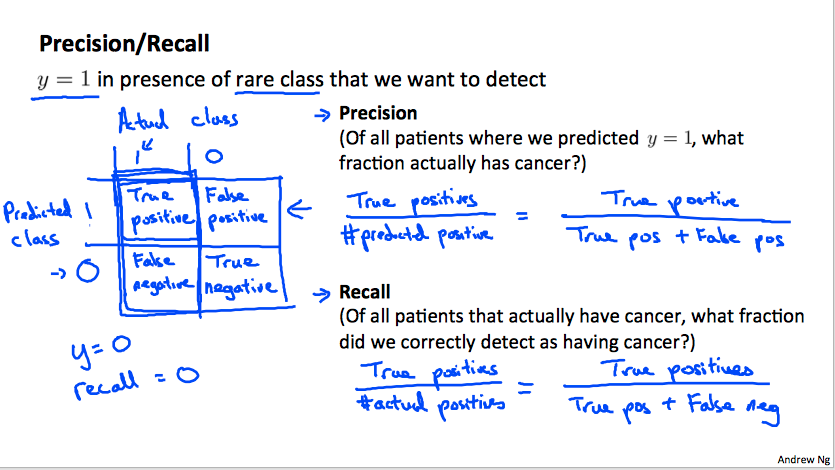• 习惯上，将$y=1$标记为不太容易出现的类别标识。(anomaly)
• precision表示预测出来为1的结果中有多少是真的1。换个理解，如果precision高，表示预测结果准确，预测出来为1的结果非常可信，因为大多数都是真的1的样例。
• recall表示标记数据集合中是1的数据有多少被预测正确。换个理解，如果recall高，表示标记集合中为1的数据基本都被预测到了，模型非常容易将数据判定为1，但可能准确度不高（太容易放行）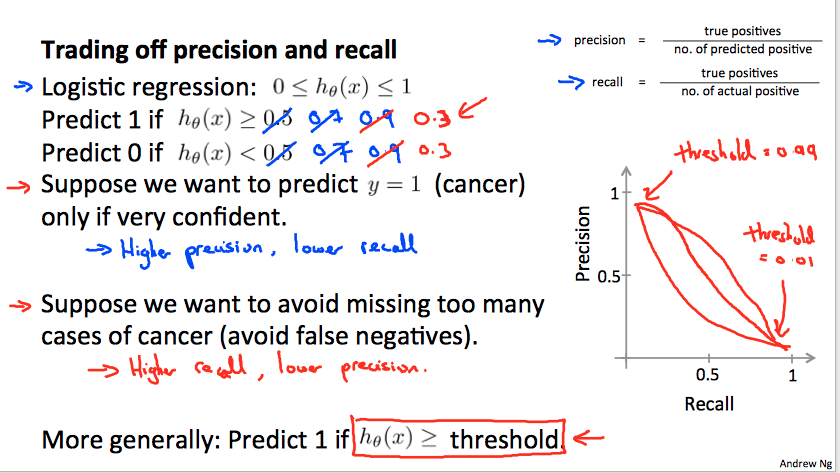• 如果希望预测的结果非常可信，设定的阈值比较高，导致precision高，但是recall低。基本上预测出来是癌症的多数是癌症，而不是让不太可能的情况预测为癌症，让病人心里难受。
• 相反，希望预测结果可以反映大多数可能是癌症的情况，让病人可后续跟进治疗，设定低阈值，导致precision低（放行太多），但是recall高。我想这也是recall的来源吧，表示还需要进一步去recall查询下，看是不是真有癌症。

$F_1 \text{Score(F Score)} = 2 \frac {PR} {P+R}$

# SVM

## Concept

SVM是一种logestic regression的变种，其第一个改动在于如何计算预测的结果和标记结果之间的代价。在logestic中，代价使用log关系来表述，函数连续，概念上定义为特定种类的概率，而SVM不使用连续函数，而是将数值截断：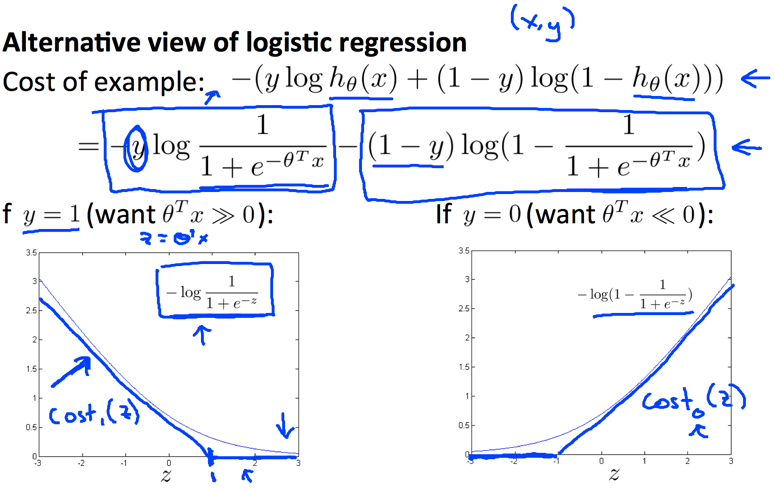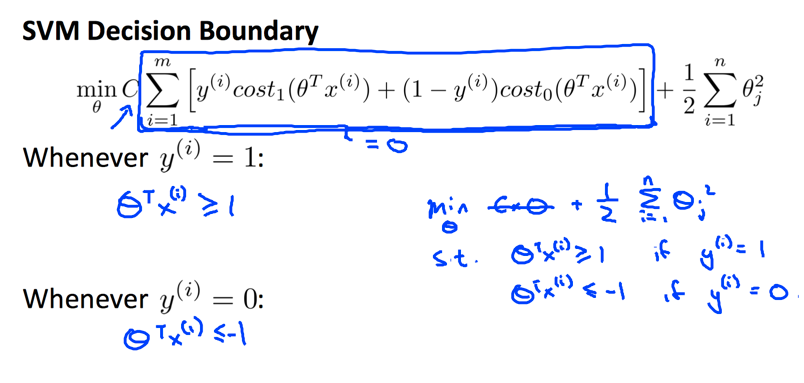• $C$参数去掉了$\frac 1 m$的计算，等价于原来的$C=\frac 1 \lambda$，所以$C$越大，越容易overfit.
• 另外，我想不计算$\frac 1 m$的原因（相比于logestic）是因为计算的代价不具备规约性。logestic的计算结果是概率规约到log函数的输出，是连续的，数据可能很小，也可能很大，所以需要$\frac 1m$进行规约，否则不同规模$m$的代价结果会完全不一样，没有可比性（不规约的话，基本上数据越多，最终的代价越大）。而这里是断层的函数，不是连续的结果，而是大是大非的结果，所以结果和规模没有关系，也就是不同规模的$m$也可以比较（相反，如果规约，可能数据越多，代价越小。试想，大多数单个数据代价是0，有几个数据代价比较大，结果一规约，代价被平均了）。

SVM也叫做large margin classification，因为其计算得到的决策边界距离数据集的边界都比较大。其实也好理解，如果划分有偏向性，或者说距离特定类别数据更近，那么另一侧数据导致的代价就会较大：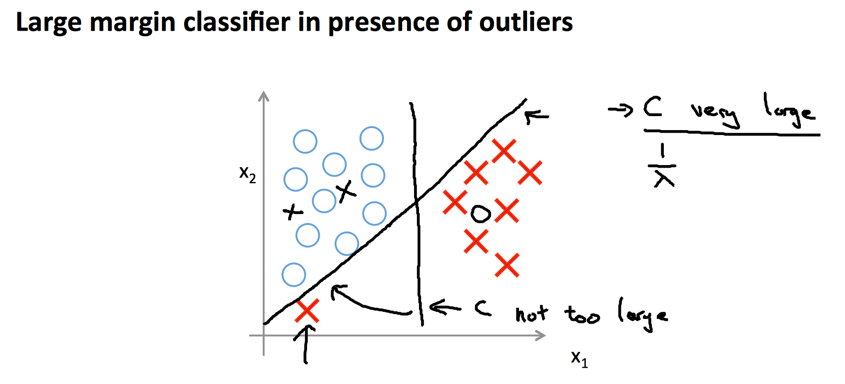$C$参数越大，划分的边界距离会变小，因为需要更多的考虑突出的节点导致的cost急剧变大，也就会更好的迎合所有节点，进而导致可能overfit。

## Kernel

SVM的另外一种形式是Non-linear，也就是特征数据组合出了非线性特征（类似于NN算法的隐含层），其核心就是引入了kernel概念。

kernel的目的是将特征集合$x$映射到另外特征集合$f$，这样就等于将特征数据进行了非线性化扩展。常用的kernel是Gaussian kernel: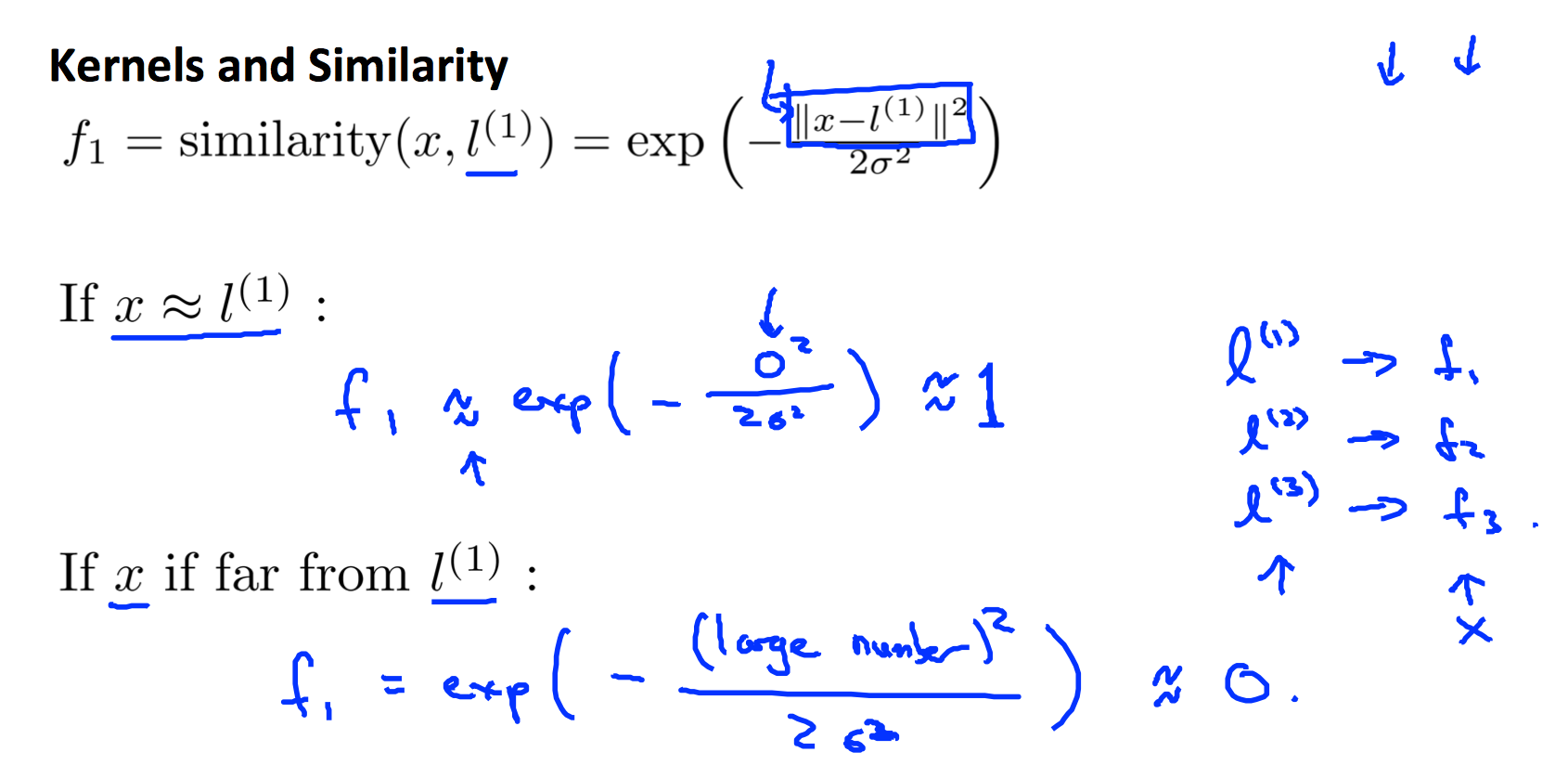• $m$很大，而$n$很小时，可能出现underfit，所以需要更多特征。
• 可以挖掘特征的地方就是已知的特征本身，因此我们要充分利用特征的相关性来构造新特征，也就是要将单个特征和全局特征联系起来考虑。
• 所以想到每一个数据和其余$m$个数据进行比较，使用相似度的方式将类似的数据集合更好的归类到一起，进而加权影响到最后的权重。（如果我们越相似，且结果也类似，那么集聚起来就成了趋势）

Gussian Kernel的图形类似一个帽子：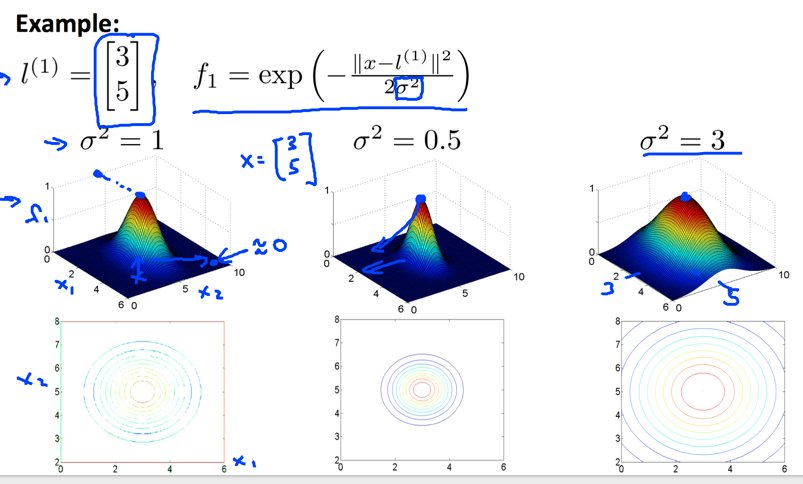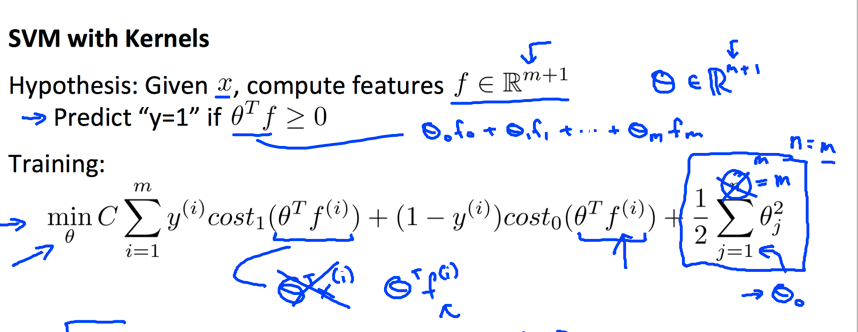## Logestic regression vs. SVMs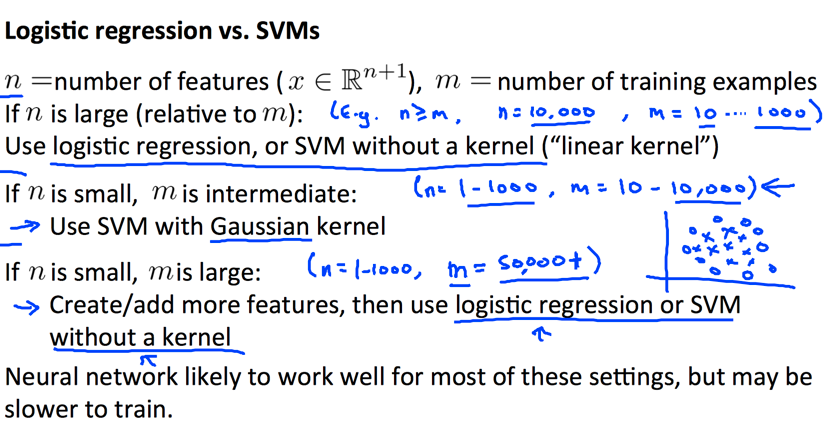• SVM使用kernel的版本是非线性模型。
• 特征数量相比于数据数量更小时，使用SVM扩展特征更好。
• 数据特别多时候，SVM计算会比较耗时，可以考虑加入更多特征，使用logestic。
• 总的说来，SVM应用场景更多，因为一般数据都没有那么多，但相比于特征又显得较多。

# K mean

K mean算法的优化方程是：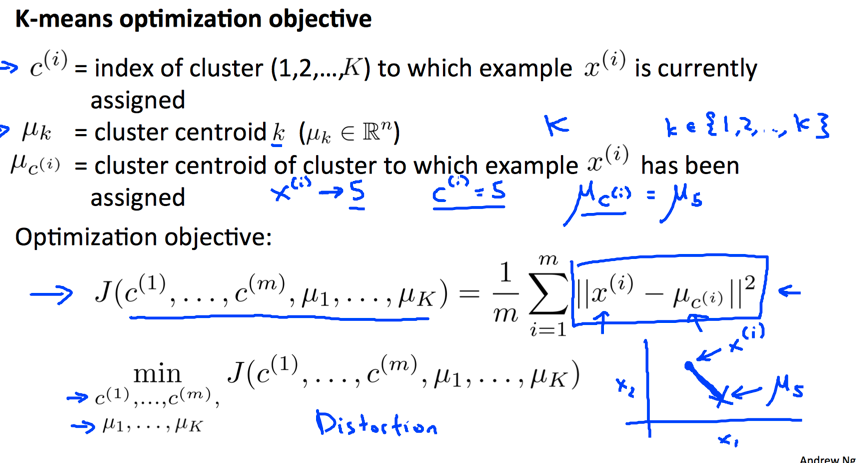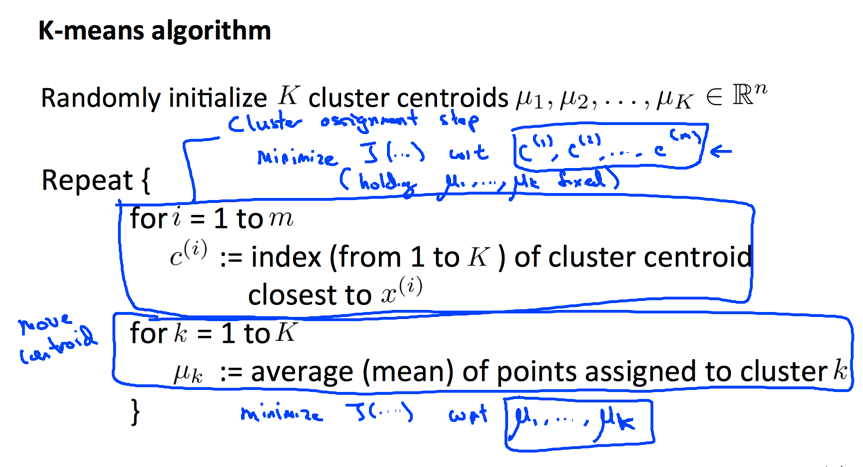• $\mu_k$确定的情况下，计算出$c^{(i)}$，也就是对应数据的类别。这个其实很容易理解，数据的类别就是距离最近的中心点标号。
• $c^{(i)}$确定情况下，也就是每个数据的判定类别确定情况下，推出使得代价$J$最小的$\mu_k$。这个也好理解，分类好数据的最小加和距离中心点就是几何中心。
• 之所以迭代算法可以解决该优化问题，是因为两个需要优化的参数存在相关性。都是在已知A的情况下明确的知道最好的B在哪里。

• 从数据中随机选择初始点（更能体验聚类的内耦合特性）
• 多次执行，取最小$J$的初始点集合。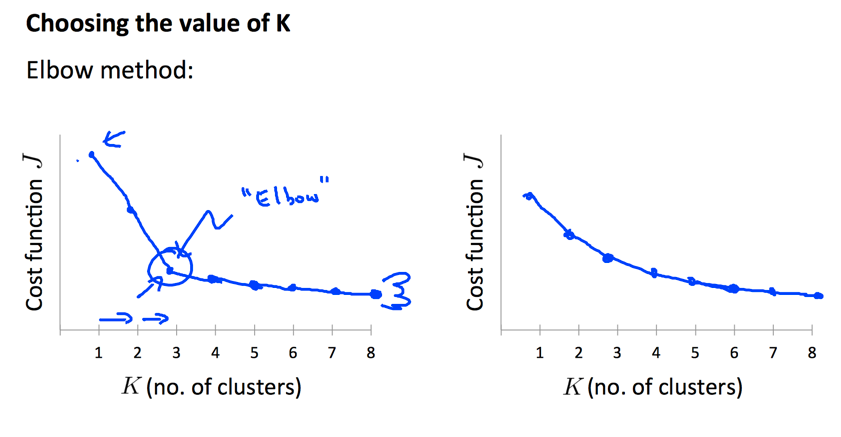# PCA

PCA主要目的在于数据降维：一方面降低了数据计算量，二方面可以用来可视化分析。试想，如果将数据压缩到2D或者3D，就可以直接画图来分析数据特性，更好地理解数据。

PCA的核心思想是将$n$维数据映射到$k$维，使得原始数据到映射平面的距离最小，这样子映射数据可以更好地反映原始数据。经过数学推导，其本质是计算原始数据集协方差矩阵的特征向量：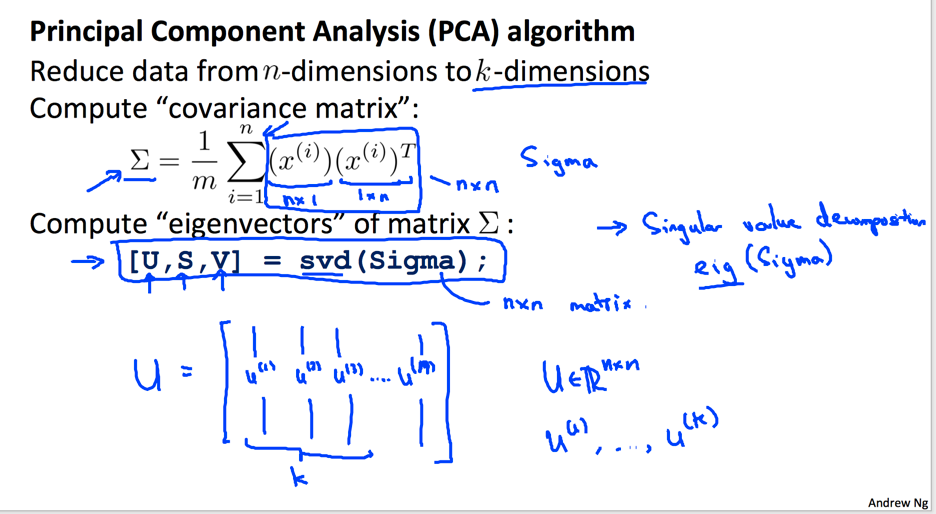$\frac {\sum_{i=1}^k S_{ii}} {\sum_{i=1}^n S_{ii}} >= 0.99$

• 使用PCA来降低overfit。PCA只是更好的近似了原始数据，但并不消灭导致过度拟合的高频信息。即使使用了PAC，原始数据的高频信息还在，并不是解决overfit的本质方法。而应该考虑增加数据量，或者加入regularization等方式。
• 不管三七二十一，一上来就用PCA进行预处理。PCA应该是在原始数据不能有效计算，或者计算量过大的时候再使用，而不是不假思索的使用。

• 使用mean normalization（处理后，均值为0），这是算法要求。
• 如果不同特征范围偏差较大，需要scale数据，归一化到$[-1, 1]$。（只要是数据之间进行相互融合计算，需要归一化，不然计算不在一个维度）

# Anomaly Dection System

Anomaly Dection是一种检测异常数据的算法，其核心思想是对各个可能的特征进行概率分析（高斯分布），然后结合起来考虑概率分布。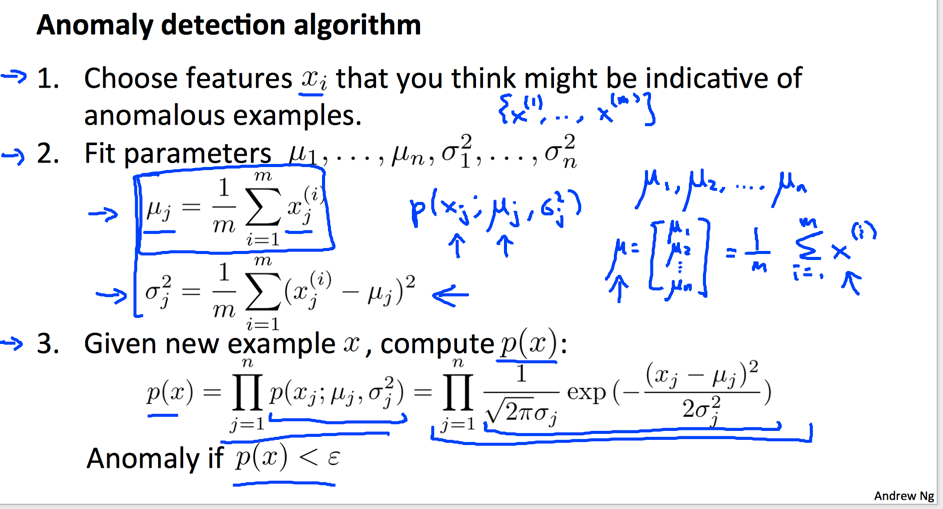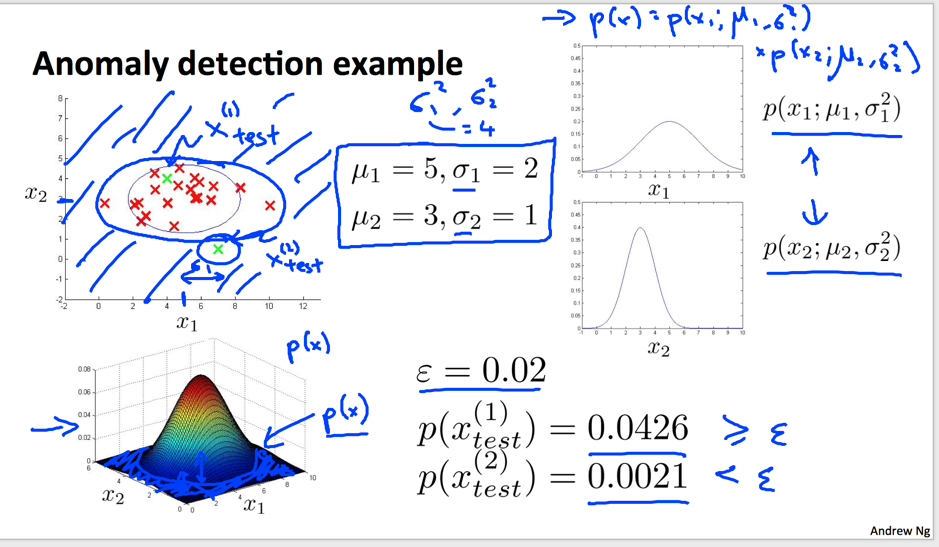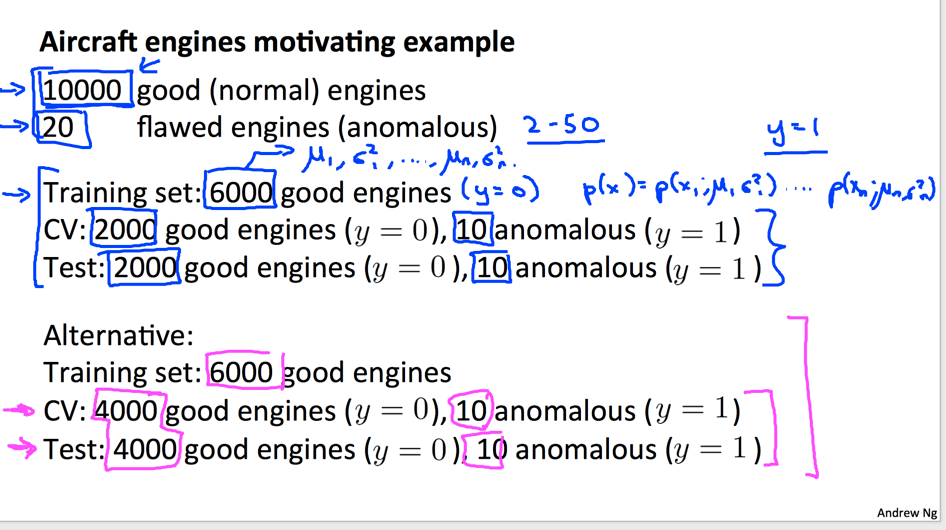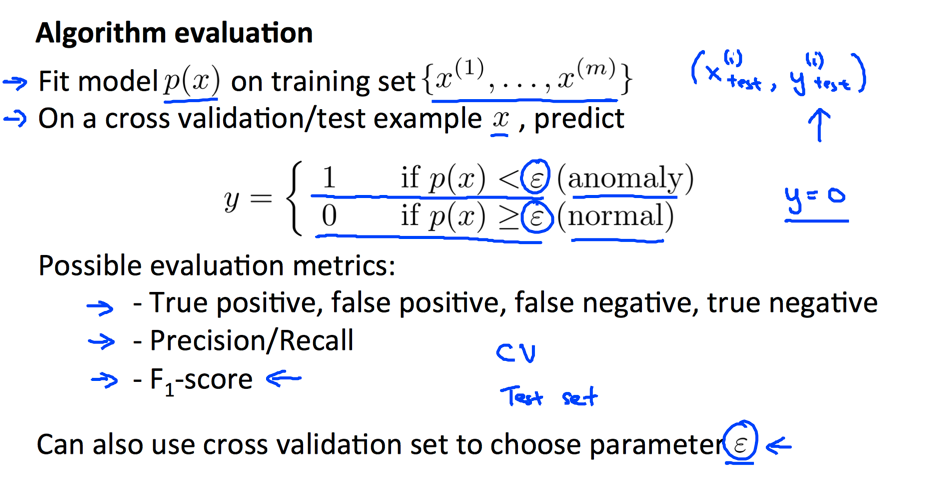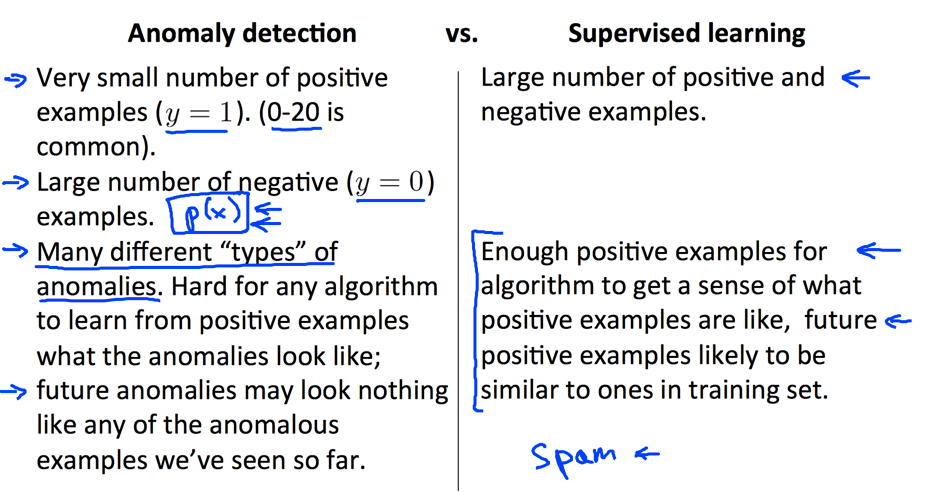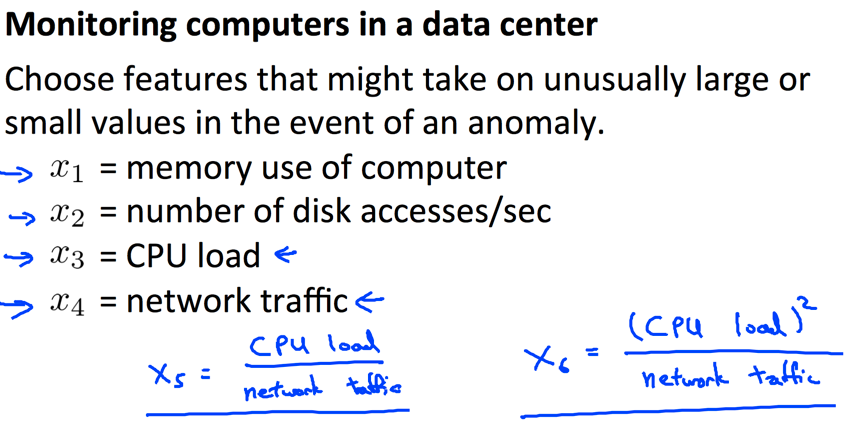# Recommendation System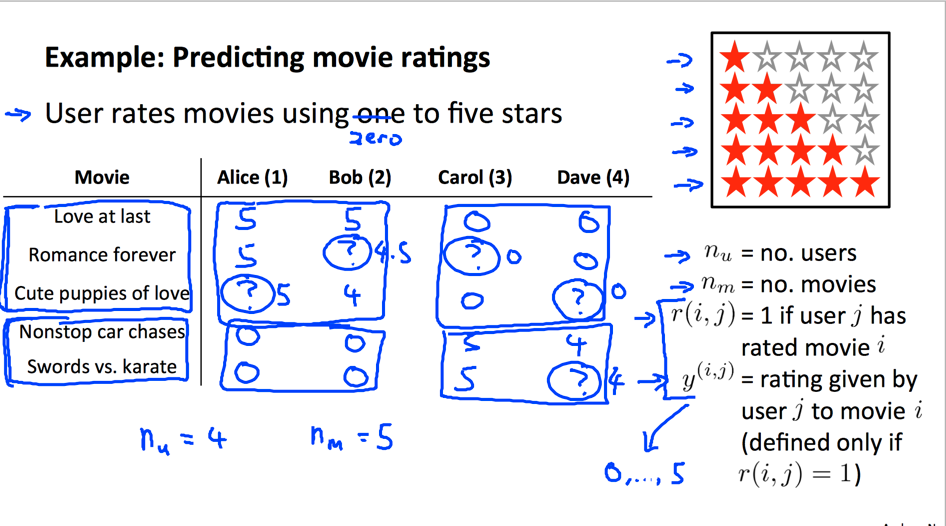• $X \in \mathbb{R}^{n_m \times n}$表示物品的特征信息，这和线性回归的输入一样。
• $Y \in \mathbb{R}^{n_m \times n_u}$表示每物品对应的每用户评价。不同的是这里，扩展了一个用户维度。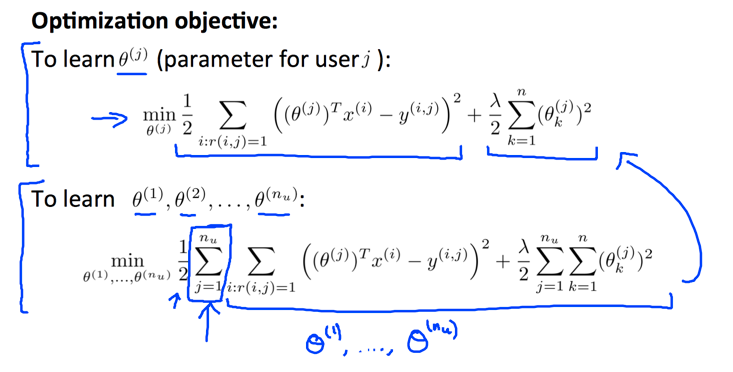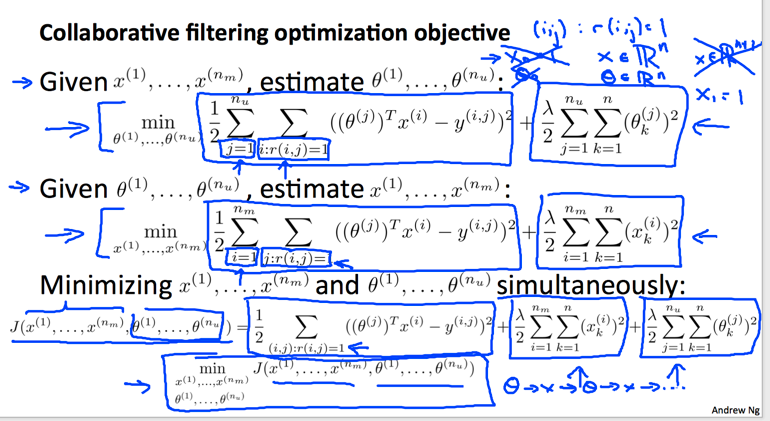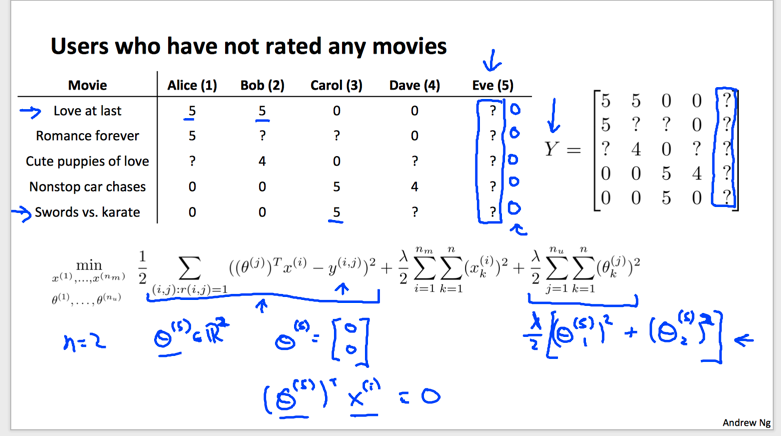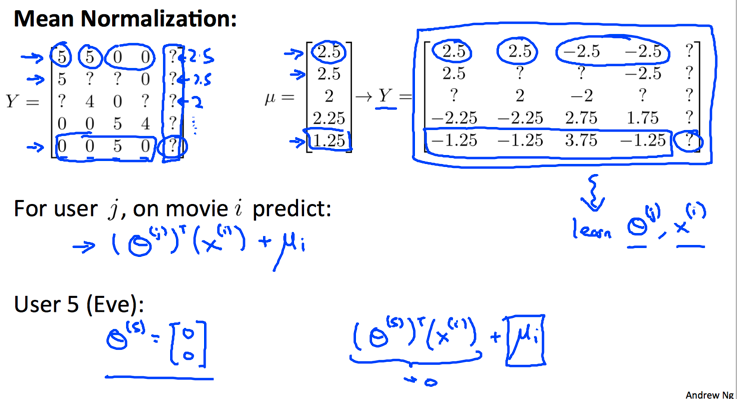# Using Large Datasets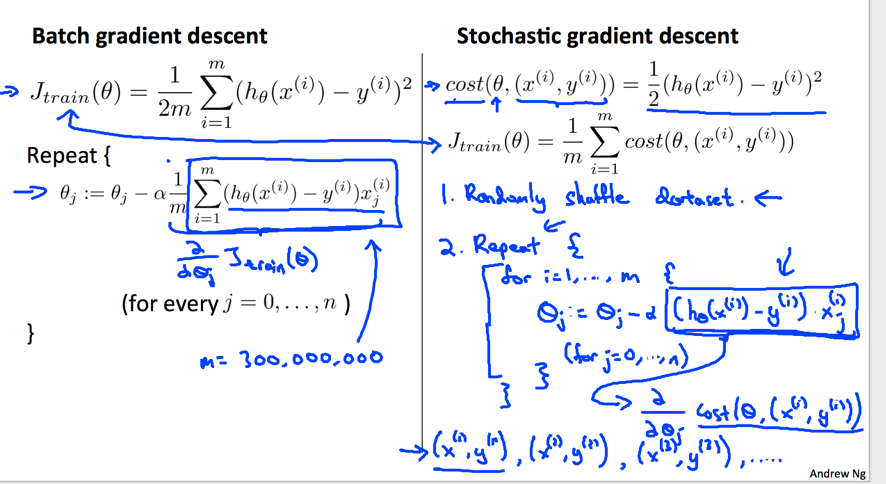• 每次只用一个数据计算偏导数，计算复杂度低。
• 相比于batch全局考虑数据(求平均)计算偏导数的方法，新方法可能并不能得到全局最优解，但随着迭代次数的增加，也会不断逼近最优解。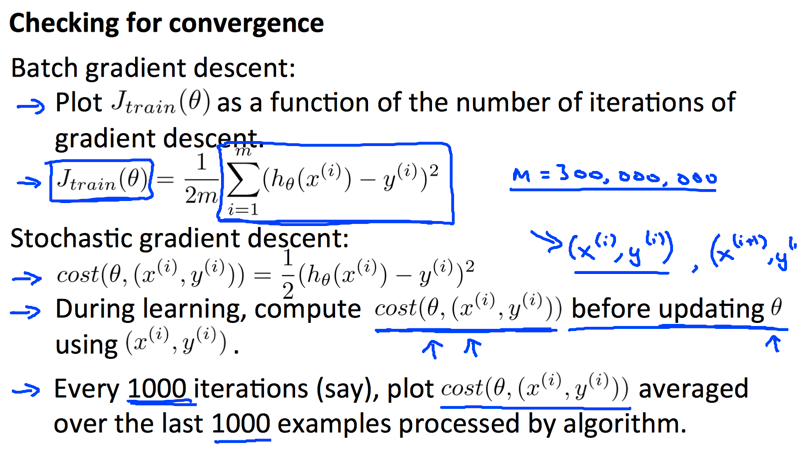stochastic模式算法非常有效的一个原因是可以处理流数据。每一次用户的点击，每一次用户的操作行为都可以做为一次训练用户偏爱程度的迭代，这种online learning有几个好处:

1. 可以处理流数据，不用保存这些海量数据。
2. 可以动态的调整计算结果。这对于模型参数可能不断变化的应用场景会更加有效。考虑，用户的口味会随着时代变化而变化。